Miscellaneous

# Dimensionless Numbers

Dimensionless numbers are frequently used in fluid mechanics equations. The basis for these dimensionless numbers is often in empirically derived correlations.

 Symbol Name Definition Significance Bi Biot Number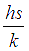.... for slab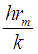.... for cylinder of sphere For a solid body, Biot number is the ratio of convective heat transfer at the surface of the body to conductive heat transfer occurring inside the body. ‘h’ is convective heat transfer coefficient. ‘s’ is characteristic length (volume/surface). ‘k’ is conductivity of the solid body. CD Drag coefficient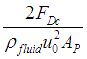Drag coefficient characterizes the drag force experienced by a body moving in a fluid. Drag coefficient is a property related to the shape of the moving object. Fo Fourier Number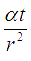Fourier number is the ratio of heat conduction rate to heat storage rate. ‘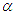’ is thermal diffusivity. ‘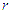’ is conduction radius and ‘ t ’ is the characteristic time. Fr Froude Number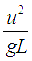Fraude number is ratio of inertial force to gravitational force. It is a measure for resistance to the motion of a body moving through water. ‘u’ is velocity of the moving object and ‘L’ is characteristic length of that object.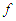Fanning friction factor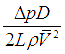The frictional pressure loss in a pipe is determined by the friction factor which is function of the Reynold’s number for the flow. Gz Graetz Number Graetz number is useful in determining the thermally developing length in flow ducts. Gz’ Graetz Number for mass transfer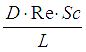Graetz number in mass transfer is useful in determining the diffusively developing length in flow ducts. Gr Grashof Number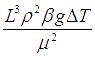Grashof number is used in fluid mechanics and heat transfer. It is the ratio of buoyancy force to viscous force. It is frequently used in studies of convection. Ma Mach Number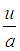Mach number for a moving object is the ratio of speed of that object moving in a fluid (u) to the speed of sound in the fluid (a).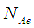Aeration Number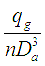Aeration number is frequently used for agitated mixing of gas and liquids.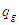is the gas volumetric flow, ‘n’ is rotational speed of the impeller, and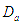is the impeller diameter.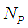Power Number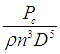Used often in correlations for agitated mixing of liquids. P is power required to rotate impeller.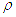is fluid density. ‘n’ is rotational speed and ‘D’ is diameter of the impeller. Power number is an equivalent of friction factor or drag coefficient for stirred liquid vessels, it is proportional to ratio of drag force acting on a unit area.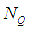Flow Number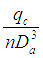For stirred liquid vessels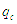is the liquid volumetric flow leaving the edges of the impeller blade, and since,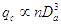, for a given impeller the Flow number is an inherent constant. Nu Nusselt Number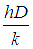In case of heat transfer occurring at the surface of a fluid, Nusselt number is the ratio of convective heat transfer to the conductive heat transfer occurring at the liquid surface. ‘h’ is convective heat transfer coefficient, ‘D’ characteristic length and k is the conductivity of fluid. Pe Peclet Numberor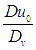Peclet number is defined to be the ratio of mass transfer occurring by advection to the mass transfer occurring by diffusion. For heat transfer, Peclet number is product of Re and Pr. For mass transfer, Peclet number is product of Re and Sc. Pr Prandtl Number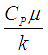Prandtl number is essentially the ratio of kinematic viscosity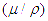to thermal diffusivity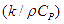. This number is only dependent on fluid and fluid state. Re Reynolds Number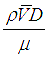Reynolds number is ratio of the inertial forces in a flowing fluid to the viscous forces acting on the fluid. Sc Schmidt NumberSimilar to the Prandtl number for heat transfer, Schmidt number in mass transfer is the ratio of kinrmatic viscosityto the mass diffusivity. Sh Sherwood Number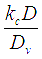Sherwood number is the mass transfer equivalent of Nusselt number from heat transfer. It is the ratio of convective mass transfer to diffusive mass transfer at the phase boundary surface.is convective mass transfer coefficient, ‘D’ is characteristic length and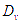is diffusivity. We Weber Number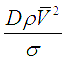It is the ratio of inertial forces acting on the fluid to the surface tension acting forces. ‘D’ is characteristic length,fluid density,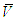fluid velocity andis surface tension acting on the fluid.